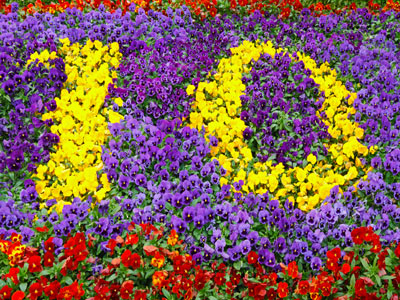What is 448 rounded to the nearest 10?

# Level 3-4 Numbers - Rounding

## Quiz about Rounding and Estimation

Rounding numbers can be useful when estimating the answers to calculations so it's an important part of KS3 Maths. Knowing whether to round a number up or down is vital. It very much depends on whether the number is above or below half way. This quiz will test you on rounding numbers up and down.

The same rules apply to rounding decimal numbers and whole ones. Just as the whole number 44 is rounded down to 40, the decimal number 4.4 is rounded down to 4. Fractions can also be rounded up or down. For example, 49 would be rounded down and 59 would be rounded up. Round the numbers to the nearest ten, hundred or thousand. For example 39 + 12 can be rounded to 40 + 10 = 50, therefore the answer will be approximately 50.

1.
What is 448 rounded to the nearest 10?
400
440
450
500
A lot of numbers in everday life are rounded
2.
What is 448 rounded to the nearest 100?
300
400
500
600
It is closer to 400 than 500
3.
What is 450 rounded to the nearest 100?
300
400
500
600
If a number is halfway between two values, round upwards
4.
What is 7,891 rounded to the nearest 1,000?
7,000
7,500
7,800
8,000
7,891 is only 109 less than 8,000 but 891 more than 7,000
5.
What is 6,691 rounded to 1 significant figure?
6
66
670
7,000
As the number has 4 columns the significant figure is 1,000
6.
What is 9.825 rounded to 2 decimal places?
9.8
9.82
9.83
9.9
Remember if it's halfway, round up!
7.
What is 277 rounded to 1 significant figure?
2
30
200
300
It's closer to 300 than to 200
8.
What is 3.98 rounded to 1 significant figure?
3
4
5
6
It's closer to 4 than to 3
9.
What is 3.114 rounded to 1 decimal place?
3
3.1
3.2
3.4
The 1 is less than 50 so is rounded down
10.
What is 1.784 rounded to 2 decimal places?
1
1.7
1.78
1.79
The 4 is less than 5 so is rounded down